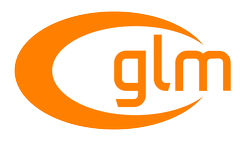0.9.9 API documentation
GLM_EXT_quaternion_common

Provides common functions for quaternion types. More...

Functions

template<typename T , qualifier Q>
GLM_FUNC_DECL qua< T, Q > conjugate (qua< T, Q > const &q)
Returns the q conjugate. More...

template<typename T , qualifier Q>
GLM_FUNC_DECL qua< T, Q > inverse (qua< T, Q > const &q)
Returns the q inverse. More...

template<typename T , qualifier Q>
GLM_FUNC_DECL vec< 4, bool, Q > isinf (qua< T, Q > const &x)
Returns true if x holds a positive infinity or negative infinity representation in the underlying implementation's set of floating point representations. More...

template<typename T , qualifier Q>
GLM_FUNC_DECL vec< 4, bool, Q > isnan (qua< T, Q > const &x)
Returns true if x holds a NaN (not a number) representation in the underlying implementation's set of floating point representations. More...

template<typename T , qualifier Q>
GLM_FUNC_DECL qua< T, Q > lerp (qua< T, Q > const &x, qua< T, Q > const &y, T a)
Linear interpolation of two quaternions. More...

template<typename T , qualifier Q>
GLM_FUNC_DECL qua< T, Q > mix (qua< T, Q > const &x, qua< T, Q > const &y, T a)
Spherical linear interpolation of two quaternions. More...

template<typename T , qualifier Q>
GLM_FUNC_DECL qua< T, Q > slerp (qua< T, Q > const &x, qua< T, Q > const &y, T a)
Spherical linear interpolation of two quaternions. More...

Detailed Description

Provides common functions for quaternion types.

Include <glm/ext/quaternion_common.hpp> to use the features of this extension.

GLM_EXT_scalar_common
GLM_EXT_vector_common
GLM_EXT_quaternion_float
GLM_EXT_quaternion_double
GLM_EXT_quaternion_exponential
GLM_EXT_quaternion_geometric
GLM_EXT_quaternion_relational
GLM_EXT_quaternion_trigonometric
GLM_EXT_quaternion_transform

Function Documentation

 GLM_FUNC_DECL qua glm::conjugate ( qua< T, Q > const & q )

Returns the q conjugate.

Template Parameters
 T A floating-point scalar type Q A value from qualifier enum
 GLM_FUNC_DECL qua glm::inverse ( qua< T, Q > const & q )

Returns the q inverse.

Template Parameters
 T A floating-point scalar type Q A value from qualifier enum
 GLM_FUNC_DECL vec<4, bool, Q> glm::isinf ( qua< T, Q > const & x )

Returns true if x holds a positive infinity or negative infinity representation in the underlying implementation's set of floating point representations.

Returns false otherwise, including for implementations with no infinity representations.

Template Parameters
 T A floating-point scalar type Q A value from qualifier enum
 GLM_FUNC_DECL vec<4, bool, Q> glm::isnan ( qua< T, Q > const & x )

Returns true if x holds a NaN (not a number) representation in the underlying implementation's set of floating point representations.

Returns false otherwise, including for implementations with no NaN representations.

/!\ When using compiler fast math, this function may fail.

Template Parameters
 T A floating-point scalar type Q A value from qualifier enum
 GLM_FUNC_DECL qua glm::lerp ( qua< T, Q > const & x, qua< T, Q > const & y, T a )

Linear interpolation of two quaternions.

The interpolation is oriented.

Parameters
 x A quaternion y A quaternion a Interpolation factor. The interpolation is defined in the range [0, 1].
Template Parameters
 T A floating-point scalar type Q A value from qualifier enum
 GLM_FUNC_DECL qua glm::mix ( qua< T, Q > const & x, qua< T, Q > const & y, T a )

Spherical linear interpolation of two quaternions.

The interpolation is oriented and the rotation is performed at constant speed. For short path spherical linear interpolation, use the slerp function.

Parameters
 x A quaternion y A quaternion a Interpolation factor. The interpolation is defined beyond the range [0, 1].
Template Parameters
 T A floating-point scalar type Q A value from qualifier enum